# Concept of average and short tricks [Method of Deviation] for CAT Exam

Concept of average and short tricks [Method of Deviation] for CAT Exam
4.7 (94.55%) 11 votes

## Concept of averages for CAT

An average is yet another essential concept for the CAT and other competitive exams. Most of us have a fair understanding of the average or mean. However, the questions from average which appear in competitive exams are tricky and often calculation intensive if we apply the standard approach.

In this article, I will be discussing the alternate approach to better understand the questions from average and also we can avoid unwanted calculation. The approach is known as the method of deviation.

So, let us begin with what we already know about average.

Simple Average (or Mean) is defined as the ratio of the sum of the quantities to the number of quantities.By Definition, Average =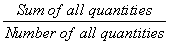Putting in symbols, X =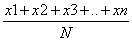## Averages Tricks: Method of deviation to find the average

The concept of an assumed mean is not new. It is widely used to reduce the calculation in finding the average in statistics where the data is huge. Here I will demonstrate the application of the assumed mean to solve some aptitude questions based on averages and weighted averages.

Let us take an example to understand the concept

Example: In a class of 30 students, the average age is 12 years. If the age of the class teacher is included, the new average age of the class becomes 13 years. Find the age of the class teacher.
Standard Approach

Applying the standard approach, the total age of the 30 students =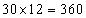years. When the class teacher is included, the new total age of the class =years. Note that the increase in the total age is because of the class teacher only. Hence the age of the class teacher = 403 – 360 = 43 years.

Deviation method

To understand deviation method, let us simulate the problem. In case of average age, assume that each student has 12 chocolates with them. The class teacher came up with few number chocolates and a redistribution of the chocolates in the class is done such that every person including the class teacher has the same number of chocolates; which results into 13 chocolates per person (the new average).

Now, it is obvious that if the class teacher comes with only 12 chocolates, the average remains the same i.e. 12 only (Because he is carrying the number of chocolates which is equal to the average number of chocolates of the group).

But here with the inclusion of class teacher, the average increases by 1. That means he came with the number of chocolates such that he gave 1 chocolate to each student so that every 30 students now has 13 chocolates. Also, at the end, he is left with 13 chocolates for himself so that including him, each member of the class is now carrying 13 chocolates.

Therefore, he came with 30 + 13 = 43 chocolates. Or the age of the class teacher is 43 years.

Let us take one more example:

Example: If a person with age 55 joins a group of 5 persons with an average age of 49 years. What will be the new average age of the group?

Solution:

Standard approach:

Total age will be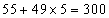. And there will be 6 persons now.

So the average will be 300/6 = 50.

Deviation approach:

Since 55 is 6 more than 49, by joining of the new person, the total will increase by 6 and number of person increases to 6; the average will increase by 1.

So, the average is 49 + 1 = 50.

## Important facts about averages

• If each number is increased/decreased by a certain quantity n, then the mean also increases or decreases by the same quantity.
• If each number is multiplied/ divided by a certain quantity n, then the mean also gets multiplied or divided by the same quantity.
• If the same value is added to half of the quantities and the same value is subtracted from other half quantities then there will not be any change in the final value of the average.

## The concept of weighted mean/average

The weighted arithmetic mean, usually denoted by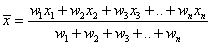,

where x1, x2, x3, …, xn are averages and w1, w2, w3, .., wn are their respective weight-ages

Example: In a class of 25 boys and 15 girls, the average heights of the two groups of boys and girls are 150 cm and 140 cm respectively. Find the average height of the class.

Standard approach:

Total weight of the group of boys =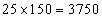and for the group of girls, the total weight =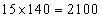Therefore, the average height of the class =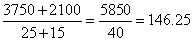Deviation Approach:

Assume that each boy and each girl is carrying 150 and 140 chocolates respectively.

We have to distribute the total chocolates such that each boy and girl must carry an equal number of chocolates.

Since each boy carries 10 chocolates more than each girl, let us take away 10 chocolates from each boy so that now each boy and girl carries 140 chocolates. The excess chocolates we have is equal to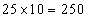. These excess chocolates are to be equally distributed among the boys and the girls. so, everyone will be getting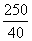=6.25 more chocolates. Hence the average of the class = 140+6.25 = 146.25 cm.